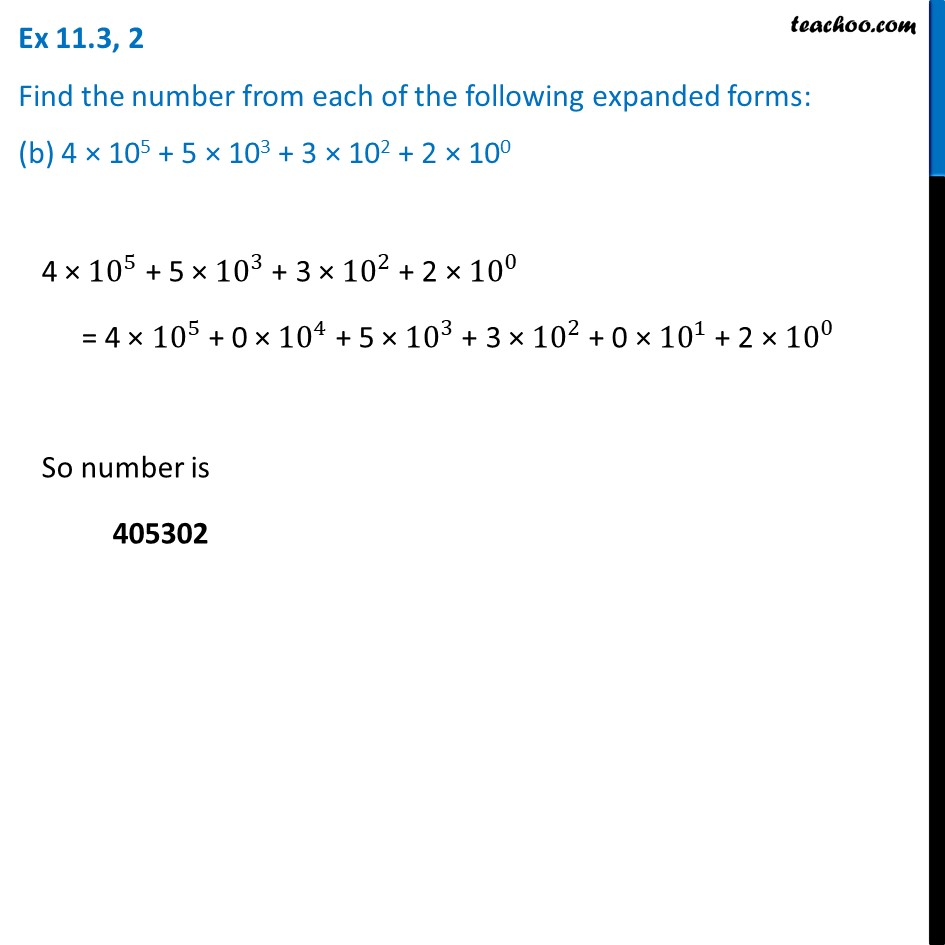Ex 11.3

Chapter 11 Class 7 Exponents and Powers
Serial order wiseLearn in your speed, with individual attention - Teachoo Maths 1-on-1 Class

### Transcript

Ex 11.3, 2 Find the number from each of the following expanded forms: (b) 4 × 105 + 5 × 103 + 3 × 102 + 2 × 100 4 × 〖10〗^5 + 5 × 〖10〗^3 + 3 × 〖10〗^2 + 2 × 〖10〗^0 = 4 × 〖10〗^5 + 0 × 〖10〗^4 + 5 × 〖10〗^3 + 3 × 〖10〗^2 + 0 × 〖10〗^1 + 2 × 〖10〗^0 So number is 405302